sbi clerk :: reasoning :: test 8

Home sbi clerk / reasoning Questions and Answers

1.  Directions(Q.1 to Q.5 ):

How many such pairs of letters are there in the word ’LABOUR’ each of which has as many letters between them in the word (in both forward and backward directions) as they have between them in the English alphabetical series ?

a

None

b

One

c

Two

d

Three

e

More than threeView AnswerDiscuss
 Answer : Option C Explanation :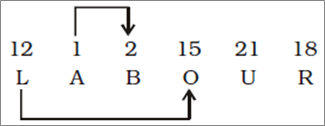2.  What should come next in the letter series given below ?
Z Z Y Z Y X Z Y X W Z Y X
W V Z Y X W V U Z Y X W V
U T Z Y X W V U T

a

U

b

Z

c

R

d

T

e

None of theseView AnswerDiscuss
 Answer : Option E Explanation :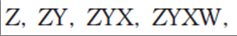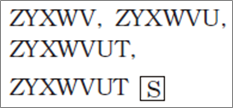3.  In a class of 20 students, Mridul’s rank is 12th from the top and Veena’s rank is 17th from the bottom. If Rohan’s rank is exactly between Mridul and Veenna’ rank, what is Rohan’s rank from the top ?

a

9th

b

8th

c

10th

d

7th

e

Cannot be determinedView AnswerDiscuss
 Answer : Option B Explanation :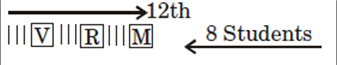Veena’s rank from the top = 20 – 17 + 1 = 4th Therefore, Rohan’s rank from the top = $\frac{{\left( {12 + 4} \right)}}{2} = 8{\rm{th}}$

4.  How many meaningful English words can be formed with the letters ’EWF’ using all the letters only once in each word ?

a

None

b

One

c

Two

d

Three

e

More than threeView AnswerDiscuss
 Answer : Option B Explanation : Meaningful Word $\Rightarrow$ FEW

5.  Four of the following five are alike in a certain way and hence form a group. Which of the following does not belong to that group ?

a

Tyre

b

Speed

c

Clutch

d

Brake

e

GearView AnswerDiscuss
 Answer : Option B Explanation : Except speed, all others are different parts of automobile.

6.  Directions(Q.6 to Q.10 ):
Study the following information carefully and answer the given questions.
Eight friends L, M, N, O, P, Q, R and S are sitting around a circle facing the centre. There are equal number of males and females in the group. No two females are immediate neighbours of each other. N is a male and N sits third to the right of R. O is a female and O is not an immediate neighbour of N. P sits second to the left of O. S sits fourth to the right of L and S is not an immediate neighbour of R. Q is a female.

What is Q’s position with respect to O ?

a

Immediate right

b

Third to the right

c

Third to the left

d

Fourth to the left

e

Fifth to the rightView AnswerDiscuss
 Answer : Option D Explanation :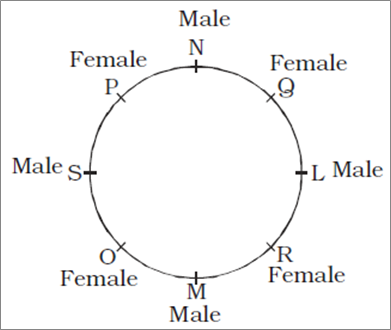Q is fourth to the left or right of O.

7.  If all the eight friends are made to sit alphabetically in the clockwise direction starting from L, positions of how many will remain unchanged (excluding L)?

a

None

b

One

c

Two

d

Three

e

FourView AnswerDiscuss
 Answer : Option B Explanation :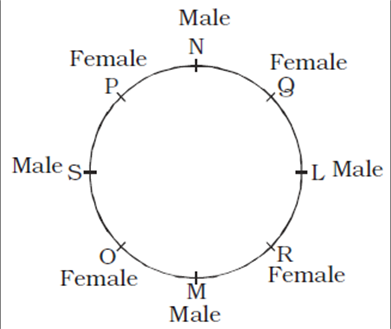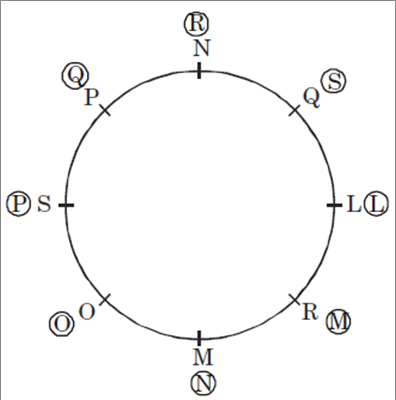8.  Which of the following is true about M ?

a

M sits third to the right of Q

b

M sits second to the right of L

c

M is a male

d

M sits to the immediate left of O

e

None of theseView AnswerDiscuss
 Answer : Option C Explanation :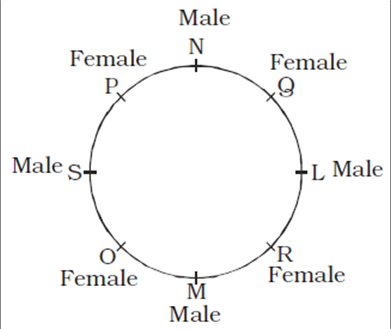M sits third to the left of Q. M sits second to the left of L. M is a male. M sits to the immediate right of O.

9.  Which of the following represent the females in the group ?

a

O, P, Q, S

b

Q, R, P, O

c

Q, L, M, O

d

O, M, Q, R

e

None of theseView AnswerDiscuss
 Answer : Option B Explanation :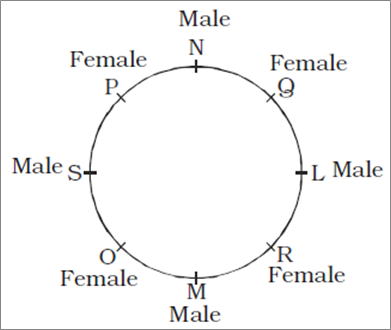The females in the group are : O, P, Q and R.

10.  Four of the following five are alike in a certain way based on their seating positions in the above arrangement and so form a group. Which is the one that does not belong to the group ?

a

LO

b

NL

c

OP

d

PQ

e

MSView AnswerDiscuss
 Answer : Option A Explanation :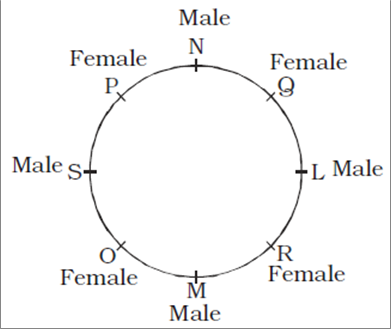Except in LO, in all others the first person is second to the right of the second person.

11.  Directions(Q.11 to Q.15 ):
In each of the questions below, two statements are given followed by two conclusions numbered I and II. You have to take the two statements to be true even if they seem to be at variance from the commonly known facts and then decide which of the given conclusions logically follows from the given statements disregarding commonly known facts and Give answer

Statements :
All tents are cabins.
All cabins are houses.
Conclusions :
I. All tents are houses.
II. All houses are tents.

a

if only conclusion I follows.

b

if only conclusion II follows.

c

if either conclusion I or conclusion II follows

d

if neither conclusion I nor conclusion II follows.

e

if both conclusions I and II follow.View AnswerDiscuss
 Answer : Option A Explanation : (i) All tents are cabins $\Rightarrow$ Universal Affirmative (A-type). (ii) Some watches are rings $\Rightarrow$ Particular Affirmative (I-type). (iii) No string is a basket.$\; \Rightarrow$ Universal Negative (E-type). (iv) Some strings are not baskets $\Rightarrow$ Particular Negative (O type).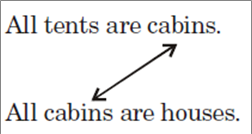A + A $\Rightarrow$ A-type of Conclusion “All tents are houses.” This is Conclusion I.

12.  Statements :
All watches are shoes.
Some watches are rings.
Conclusions :
I. Some rings are shoes.
II. All rings are shoes.

a

if only conclusion I follows.

b

if only conclusion II follows.

c

if either conclusion I or conclusion II follows

d

if neither conclusion I nor conclusion II follows.

e

if both conclusions I and II follow.View AnswerDiscuss
 Answer : Option A Explanation : (i) All tents are cabins $\Rightarrow$ Universal Affirmative (A-type). (ii) Some watches are rings $\Rightarrow$ Particular Affirmative (I-type). (iii) No string is a basket.$\; \Rightarrow$ Universal Negative (E-type). (iv) Some strings are not baskets $\Rightarrow$ Particular Negative (O type).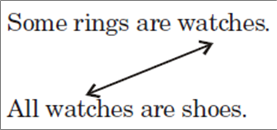I + A $\Rightarrow$ I-type of Conclusion “Some rings are shoes.” This is Conclusion I.

13.  Statements :
All purses are strings.
Conclusions :
I. No purse is a basket.
II. At least some purses are baskets.

a

if only conclusion I follows.

b

if only conclusion II follows.

c

if either conclusion I or conclusion II follows

d

if neither conclusion I nor conclusion II follows.

e

if both conclusions I and II follow.View AnswerDiscuss
 Answer : Option A Explanation : (i) All tents are cabins $\Rightarrow$ Universal Affirmative (A-type). (ii) Some watches are rings $\Rightarrow$ Particular Affirmative (I-type). (iii) No string is a basket.$\; \Rightarrow$ Universal Negative (E-type). (iv) Some strings are not baskets $\Rightarrow$ Particular Negative (O type).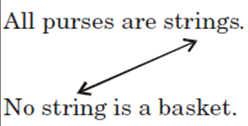A + E $\Rightarrow$ E-type of Conclusion “No purse is a basket.” This is Conclusion I.

14.  Statements :
Some weathers are rains.
Some summers are weathers.
Conclusions :
I. At least some summers are rains.
II. At least some weathers are summers.

a

if only conclusion I follows.

b

if only conclusion II follows.

c

if either conclusion I or conclusion II follows

d

if neither conclusion I nor conclusion II follows.

e

if both conclusions I and II follow.View AnswerDiscuss
 Answer : Option B Explanation : (i) All tents are cabins $\Rightarrow$ Universal Affirmative (A-type). (ii) Some watches are rings $\Rightarrow$ Particular Affirmative (I-type). (iii) No string is a basket.$\; \Rightarrow$ Universal Negative (E-type). (iv) Some strings are not baskets $\Rightarrow$ Particular Negative (O type). Both the Premises are Particular Affirmative (I-type). No Conclusion follows from the two Particular Premises. Conclusion II is Converse of the second Premise.

15.  Statements :
Some glasses are tablets.
All machines are tablets.
Conclusions :
I. All tablets being machines is a possibility.
II. All machines being glasses is a possibility.

a

if only conclusion I follows.

b

if only conclusion II follows.

c

if either conclusion I or conclusion II follows

d

if neither conclusion I nor conclusion II follows.

e

if both conclusions I and II follow.View AnswerDiscuss
 Answer : Option D Explanation : (i) All tents are cabins $\Rightarrow$ Universal Affirmative (A-type). (ii) Some watches are rings $\Rightarrow$ Particular Affirmative (I-type). (iii) No string is a basket.$\; \Rightarrow$ Universal Negative (E-type). (iv) Some strings are not baskets $\Rightarrow$ Particular Negative (O type).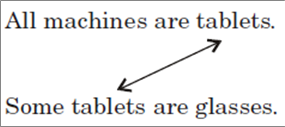A + I $\Rightarrow$ No Conclusion